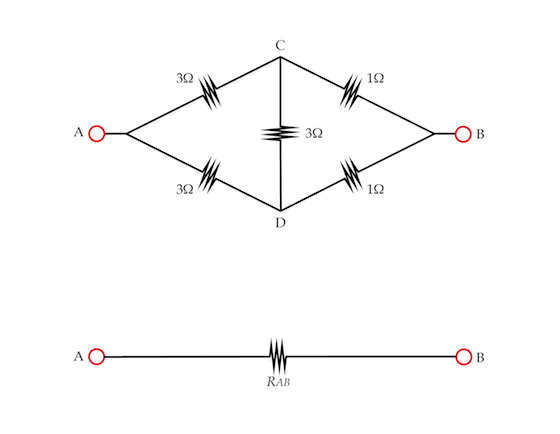# Neither series nor parallel circuit

Five resistors are connected as shown below.Note that the resistors are neither connected in series nor in parallel. However, it turns out that the set of resistors is equivalent to a single resistor $R_{AB}$. Determine the resistance in Ohms $R_{AB}$.

×